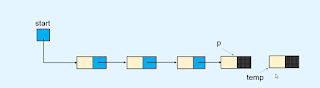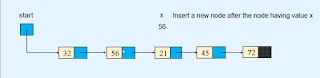Insertion - in a linked list to insert a node at any position like at the end, first, or in between the nodes, we need a reference to the node of the list.

so first we need to find the reference to the node of the list. and if you don't know how to find the reference to the node of the list then first read this post.
After completing this tutorial you are able to
1. Insert the node beginning of the list
2. Insert the node in an empty list
3. Insert the node at the end of the list
4. Insert the node in between the nodes
5. Insert the node after a node
6. Insert the node before a node
7. Insert the node at a given position

## Insert the node beginning of the list

to insert a node at the beginning of the list first we need to allocate a new node called temp as you see in the image given below.

after that, we point the linked part of the new node to the first node of the list. and then we point the start variable to the temp node. so it will become the first node of the list.

Note: the process and steps we follow are very important because if we reverse the order of steps to insert the node then we stuck in the infinite loop of the list.

```def insert_in_beginning(self, data):
temp = Node(data)
self.start = temp```

## Insert the node in an empty list

in an empty we don't have any node. so till now, our list is empty the reference to the first node is null or None.

first, we allocate a new node called temp. and then we point the reference of the node to the start variable.

```def insert_at_end(self, data):
temp = Node(data)
if self.start is None:
self.start = temp```

## Insert the node at the end of the list

to insert a node at the end of the list first we need a reference to the last node of the list. after finding the reference to the last node of the list. first, we allocate a new node called temp.```def insert_at_end(self, data):
temp = Node(data)
if self.start is None:
self.start = temp
return
p = self.start
while p.link is not None:

## Insert the node in between the nodes.

to insert a node in the between the nodes we need a reference to the node after we are going to insert the node. as you see in the image. and we allocate a new node called temp.and then we store the reference of the temp node into the p node.

## Insert a node after a node

for example, we need to insert the node after the node that having value x=56 then first we need to find the reference to the node that has the particular value.and then we allocate a new node called temp.

then we store the reference of the newly allocated node into the p node.```def insert_after(self, data, x):
p = self.start
while p is not None:
if p.info == x:
break        p = p.link

if p is None:
print(x, "not present in the list")
else:
temp = Node(data)

## Insert a node before a node

as if we want to insert the node before a node that has value x. for example, we need to insert the node before the node that has value x=45. then first we need to find the reference to the predecessor of the node that has value x.

```def insert_before(self, data, x):
# If list is empty    if self.start is None:
print("List is empty")
return    # x is in first node , ew node is to be inserted before first node    if x == self.start.info:
temp = Node(data)
self.start = temp
return
# Find reference to predecessor of node contanining x    p = self.start
while p.link is not None:
if p.link.info == x:
break        p = p.link

if p.link is None:
print(x, " not present in the list")
else:
temp = Node(data)

## Insert a node at a given position.

like if we want to insert a node at the kth position in the list. then first we need to find the reference to the node at the k-1 position.

for example, if we want to insert the node at the position k=4 then we find the reference to the node at the 3rd position. as you see in the image given below.

```def insert_at_position(self, data, k):
if k == 1:
temp = Node(data)
self.start = temp
return
p = self.start
i = 1    while i < k - 1 and p is not None:
i += 1
if p is None:
print("You can insert only upto position", i)
else:
temp = Node(data)

#### Python program for traversing in the linked list.

```class Node:

def __init__(self, value):
self.info = value

def __init__(self):
self.start = None
def display_list(self):
if self.start is None:
print("List is empty")
return        else:
print("List is :   ")
p = self.start
while p is not None:
print(p.info, " ", end=' ')
print()

def count_nodes(self):
p = self.start
n = 0        while p is not None:
n += 1            p = p.link
print("Number of nodes in the list = ", n)

def search(self):
position = 1        p = self.start
while p is not None:
if p.info == x:
print(x, " is at position ", position)
return True            position += 1            p = p.link
else:
return False
def insert_in_beginning(self, data):
temp = Node(data)
self.start = temp

def insert_at_end(self, data):
temp = Node(data)
if self.start is None:
self.start = temp
return
p = self.start
while p.link is not None:

def create_list(self):
n = int(input("Enter the number of nodes  :  "))
if n == 0:
return        for i in range(n):
data = int(input("Enter the element to be inserted : "))
self.insert_at_end(data)

def insert_after(self, data, x):
p = self.start
while p is not None:
if p.info == x:
break            p = p.link

if p is None:
print(x, "not present in the list")
else:
temp = Node(data)

def insert_before(self, data, x):
# If list is empty        if self.start is None:
print("List is empty")
return
if x == self.start.info:
temp = Node(data)
self.start = temp
return

p = self.start
while p.link is not None:
if p.link.info == x:
break            p = p.link

if p.link is None:
print(x, " not present in the list")
else:
temp = Node(data)

def insert_at_position(self, data, k):
if k == 1:
temp = Node(data)
self.start = temp
return
p = self.start
i = 1        while i < k - 1 and p is not None:
i += 1
if p is None:
print("You can insert only upto position", i)
else:
temp = Node(data)

def delete_node(self, x):

if self.start is None:
print("List is empty")

if self.start.info == x:
return
p = self.start
while p.link is not None:
if p.link.info == x:
break            p = p.link

if p.link is None:
print("Element ", x , "not in list")
else:

def delete_first_node(self):
if self.start is None:
return        self.start = self.start.link

def delete_last_node(self):

if self.start is None:
return
if self.start.link is None:
self.start = None            return
p = self.start
def reverse_list(self):

prev = None        p = self.start
while p is not None:
prev = p
p = next
self.start = prev

def bubble_sort_exdata(self):

end = None
while end != self.start.link:
p = self.start
while p.link != end:
if p.info > q.info:
p.info, q.info = q.info, p.info
end = p

end = None
while end != self.start.link:
r = p = self.start
while p.link != end:
if p.info > q.info :
if p != self.start:
else:
self.start = q
p,q = q,p
r = p
end = p

def has_cycle(self):
if self.find_cycle() is None:
return False        else:
return True
def find_cycle(self):
if self.start is None or self.start.link is None:
return None
slowR = self.start
fastR = self.start

while fastR is not None and fastR.link is not None:
if slowR == fastR:
return slowR
return None
def remove_cycle(self):
c = self.find_cycle()
if c is None:
return        print("Node at which the cycle was detected is ", c.info)

p = c
q = c
len_cycle = 0
while True:
len_cycle+=1            q = q.link
if p == q:
break        print("Length of cycle is :", len_cycle)

len_rem_list = 0        p = self.start
while p != q:
len_rem_list+=1            p = p.link

print("Number of nodes not included in the cycle are : ", len_rem_list)
length_list = len_cycle + len_rem_list
print("Length of the list is : ", length_list)

p = self.start
for i in range(length_list-1):

def insert_cycle(self, x):
if self.start is None:
return        p = self.start
px = None        prev = None
while p is not None:
if p.info == x:
px = p
prev = p

if px is not None:
else:
print(x, " not present in list")

def merge2(self, list2):
merge_list.start = self._merge2(self.start, list2.start)
return merge_list

def _merge2(self, p1, p2):
if p1.info <= p2.info:
startM = p1
else:
startM = p2
pM = startM

while p1 is not None and p2 is not None:
if p1.info <= p2.info:
else:

if p1 is None:
else:

return startM

def merge_sort(self):
self.start = self._merge_sort_rec(self.start)

def _merge_sort_rec(self, list_start):

if list_start is None or list_start.link is None:
return list_start

start1 = list_start
start2 = self._divide_list(list_start)
start1 = self._merge_sort_rec(start1)
start2 = self._merge_sort_rec(start2)
startM = self._merge2(start1, start2)

return startM

def _divide_list(self, p):
while q is not None and q.link is not None:
p.link = None        return start2

##########################

list.create_list()

while True:
print("1. Display list")
print("2. Count the number of nodes")
print("3. Search for an element")
print("4. Insert in empty list/insert in beginning of the list")
print("5. Insert a node at the end of the list")
print("6. Insert a node after a specified node")
print("7. Insert a node before a specified node")
print("8. Insert a node at a given position")
print("9. Delete first node")
print("10. Delete last node")
print("11. Delete any node")
print("12. Reverse the list")
print("13. Bubble sort by exchanging data")
print("14. Bubble sort by exchanging links")
print("15. Merge sort")
print("16. Insert Cycle")
print("17. Detect Cycle")
print("18. Remove Cycle")
print("19. Quite")

option = int(input("Enter your choice"))

if option == 1:
list.display_list()
elif option == 2:
list.count_nodes()
elif option == 3:
data = int(input("Enter the element to be searched : "))
list.search(data)
elif option == 4:
data = int(input("Enter the element to be inserted : "))
list.insert_in_beginning(data)
elif option == 5:
data = int(input("Enter the element to be inserted : "))
list.insert_at_end(data)
elif option == 6:
data = int(input("Enter the element to be inserted : "))
x = int(input("Enter the element after which to insert : "))
list.insert_after(data, x)
elif option == 7:
data = int(input("Enter the element to be inserted : "))
x = int(input("Enter the element before which to insert : "))
list.insert_before(data, x)
elif option == 8:
data = int(input("Enter the element to be inserted : "))
k = int(input("Enter the position at which to insert : "))
list.insert_at_position(data, k)
elif option == 9:
list.delete_first_node()
elif option == 10:
list.delete_last_node()
elif option == 11:
data = int(input("Enter the element to be deleted : "))
list.delete_node(data)
elif option == 12:
list.reverse_list()
elif option == 13:
list.bubble_sort_exdata()
elif option == 14:
elif option == 15:
list.merge_sort()
elif option == 16:
data = int(input("Enter the element at which the cycle has to be inserted : "))
list.insert_cycle(data)
elif option == 17:
if list.has_cycle():
print("List has a cycle")
else:
print("List does not have a cycle")
elif option == 18:
list.remove_cycle()
elif option == 19:
break    else:
print("Wrong option")
print()```